# UNIT 1 (1).pptx

1 Jun 2023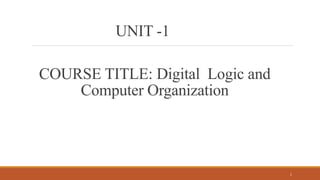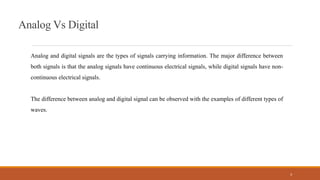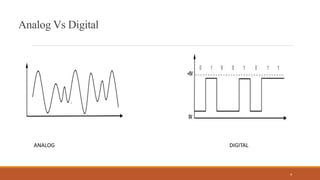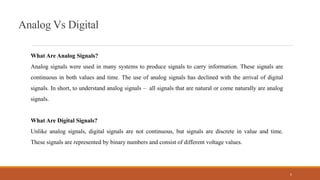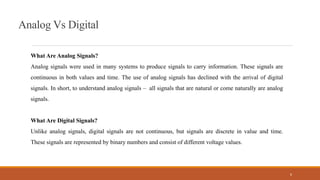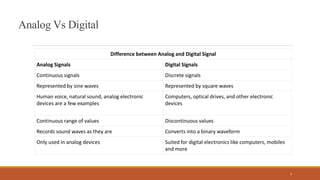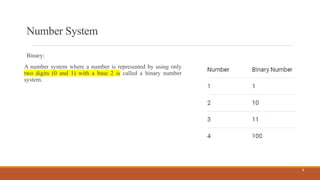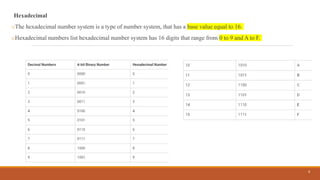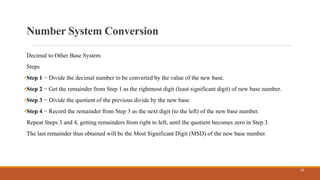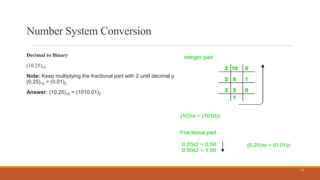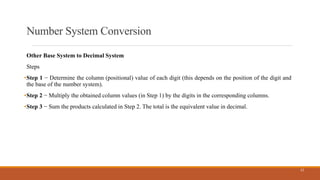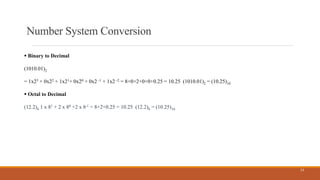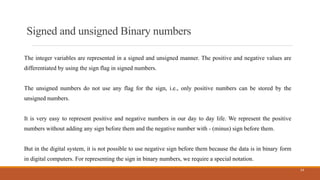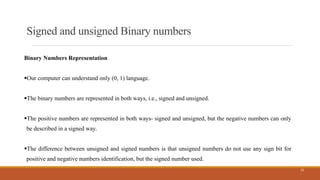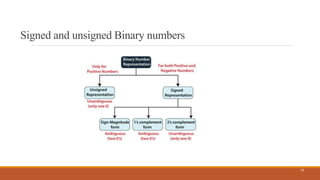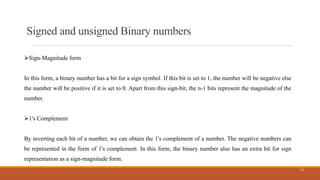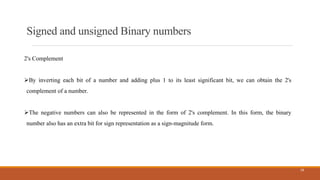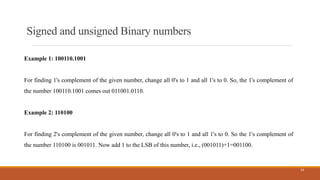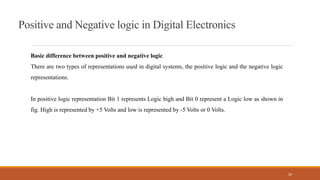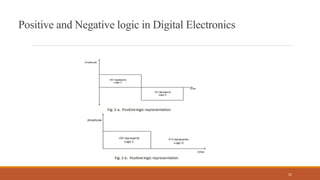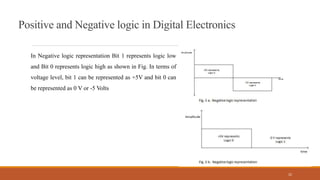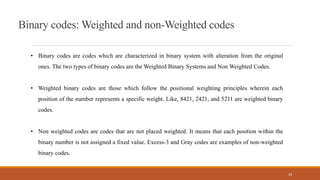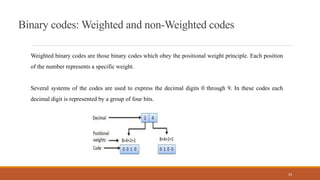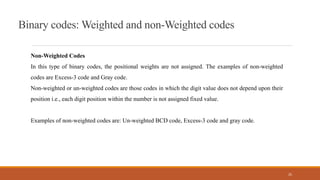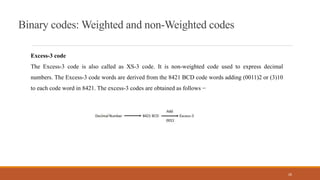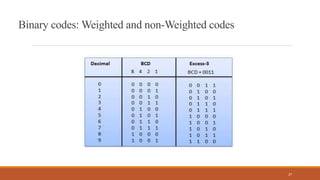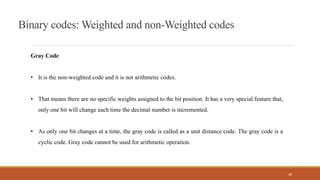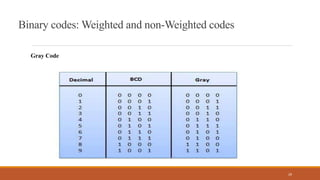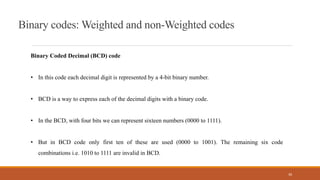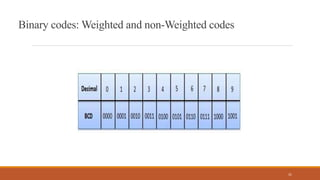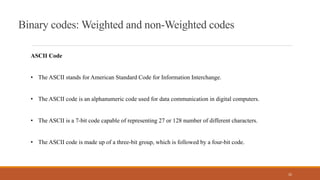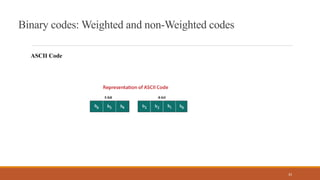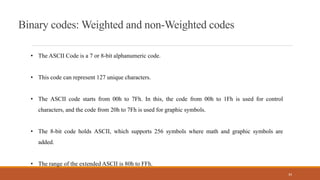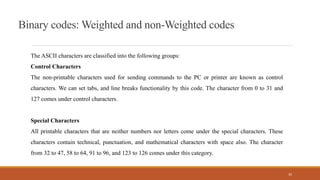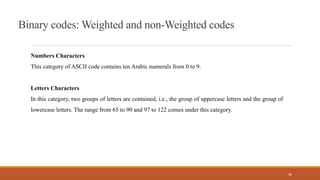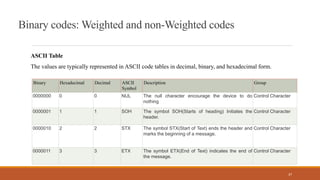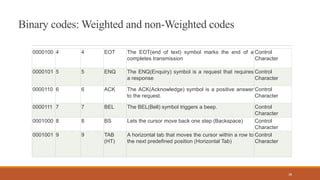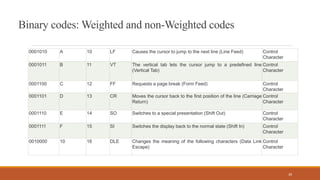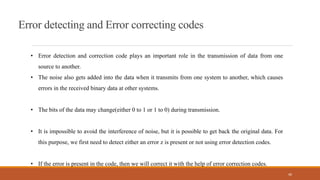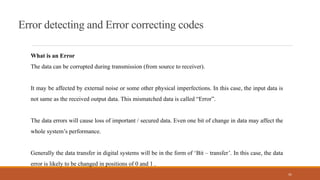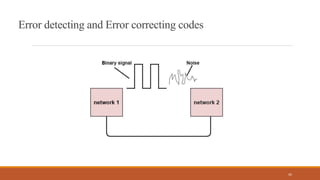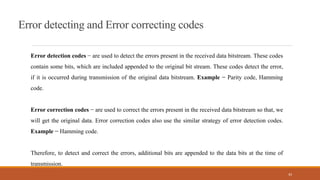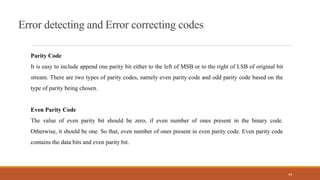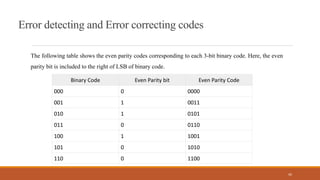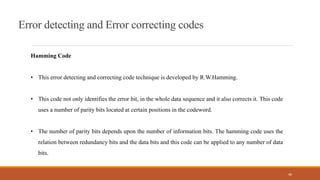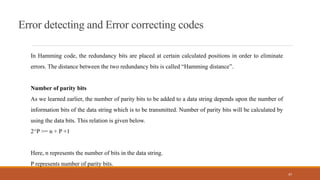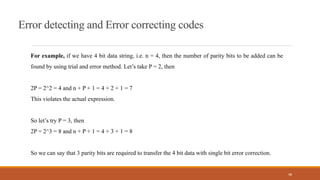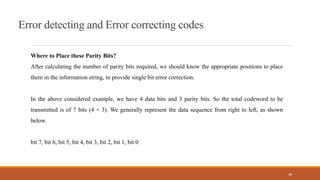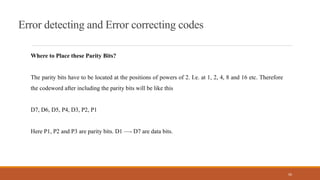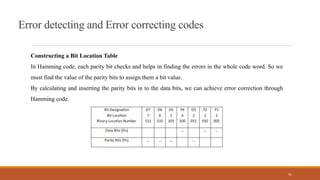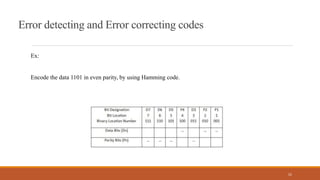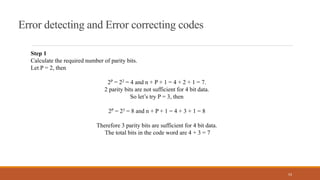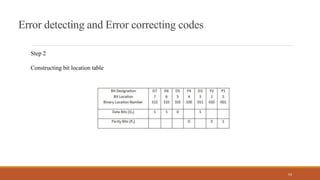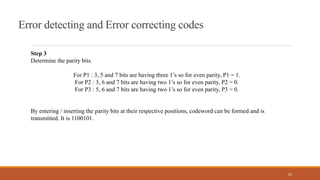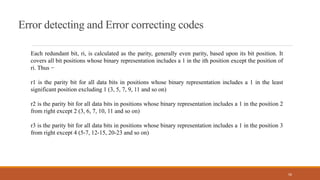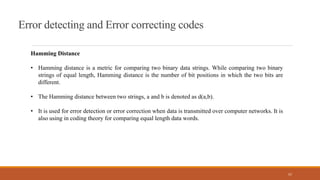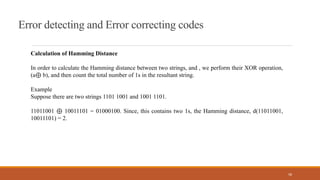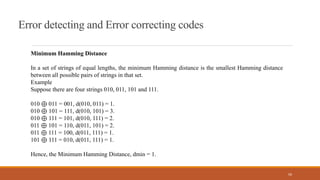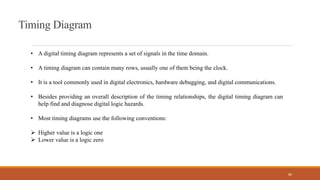1 sur 60

### UNIT 1 (1).pptx

• 1. COURSE TITLE: Digital Logic and Computer Organization 1 UNIT -1
• 3. 3 Analog Vs Digital Analog and digital signals are the types of signals carrying information. The major difference between both signals is that the analog signals have continuous electrical signals, while digital signals have non- continuous electrical signals. The difference between analog and digital signal can be observed with the examples of different types of waves.
• 5. 5 Analog Vs Digital What Are Analog Signals? Analog signals were used in many systems to produce signals to carry information. These signals are continuous in both values and time. The use of analog signals has declined with the arrival of digital signals. In short, to understand analog signals – all signals that are natural or come naturally are analog signals. What Are Digital Signals? Unlike analog signals, digital signals are not continuous, but signals are discrete in value and time. These signals are represented by binary numbers and consist of different voltage values.
• 6. 6 Analog Vs Digital What Are Analog Signals? Analog signals were used in many systems to produce signals to carry information. These signals are continuous in both values and time. The use of analog signals has declined with the arrival of digital signals. In short, to understand analog signals – all signals that are natural or come naturally are analog signals. What Are Digital Signals? Unlike analog signals, digital signals are not continuous, but signals are discrete in value and time. These signals are represented by binary numbers and consist of different voltage values.
• 7. 7 Analog Vs Digital Difference between Analog and Digital Signal Analog Signals Digital Signals Continuous signals Discrete signals Represented by sine waves Represented by square waves Human voice, natural sound, analog electronic devices are a few examples Computers, optical drives, and other electronic devices Continuous range of values Discontinuous values Records sound waves as they are Converts into a binary waveform Only used in analog devices Suited for digital electronics like computers, mobiles and more
• 8. Number System Binary: A number system where a number is represented by using only two digits (0 and 1) with a base 2 is called a binary number system. 8
• 9. Hexadecimal oThe hexadecimal number system is a type of number system, that has a base value equal to 16. oHexadecimal numbers list hexadecimal number system has 16 digits that range from 0 to 9 and A to F. 9
• 10. Number System Conversion Decimal to Other Base System Steps •Step 1 − Divide the decimal number to be converted by the value of the new base. •Step 2 − Get the remainder from Step 1 as the rightmost digit (least significant digit) of new base number. •Step 3 − Divide the quotient of the previous divide by the new base. •Step 4 − Record the remainder from Step 3 as the next digit (to the left) of the new base number. Repeat Steps 3 and 4, getting remainders from right to left, until the quotient becomes zero in Step 3. The last remainder thus obtained will be the Most Significant Digit (MSD) of the new base number. 10
• 11. Number System Conversion Decimal to Binary (10.25)10 Note: Keep multiplying the fractional part with 2 until decimal part 0.00 is obtained. (0.25)10 = (0.01)2 Answer: (10.25)10 = (1010.01)2 11
• 12. Number System Conversion Other Base System to Decimal System Steps •Step 1 − Determine the column (positional) value of each digit (this depends on the position of the digit and the base of the number system). •Step 2 − Multiply the obtained column values (in Step 1) by the digits in the corresponding columns. •Step 3 − Sum the products calculated in Step 2. The total is the equivalent value in decimal. 12
• 13. Number System Conversion  Binary to Decimal (1010.01)2 = 1x23 + 0x22 + 1x21+ 0x20 + 0x2 -1 + 1x2 -2 = 8+0+2+0+0+0.25 = 10.25 (1010.01)2 = (10.25)10  Octal to Decimal (12.2)8 1 x 81 + 2 x 80 +2 x 8-1 = 8+2+0.25 = 10.25 (12.2)8 = (10.25)10 13
• 14. Signed and unsigned Binary numbers The integer variables are represented in a signed and unsigned manner. The positive and negative values are differentiated by using the sign flag in signed numbers. The unsigned numbers do not use any flag for the sign, i.e., only positive numbers can be stored by the unsigned numbers. It is very easy to represent positive and negative numbers in our day to day life. We represent the positive numbers without adding any sign before them and the negative number with - (minus) sign before them. But in the digital system, it is not possible to use negative sign before them because the data is in binary form in digital computers. For representing the sign in binary numbers, we require a special notation. 14
• 15. Signed and unsigned Binary numbers Binary Numbers Representation Our computer can understand only (0, 1) language. The binary numbers are represented in both ways, i.e., signed and unsigned. The positive numbers are represented in both ways- signed and unsigned, but the negative numbers can only be described in a signed way. The difference between unsigned and signed numbers is that unsigned numbers do not use any sign bit for positive and negative numbers identification, but the signed number used. 15
• 16. Signed and unsigned Binary numbers 16
• 17. Signed and unsigned Binary numbers Sign-Magnitude form In this form, a binary number has a bit for a sign symbol. If this bit is set to 1, the number will be negative else the number will be positive if it is set to 0. Apart from this sign-bit, the n-1 bits represent the magnitude of the number. 1's Complement By inverting each bit of a number, we can obtain the 1's complement of a number. The negative numbers can be represented in the form of 1's complement. In this form, the binary number also has an extra bit for sign representation as a sign-magnitude form. 17
• 18. Signed and unsigned Binary numbers 2's Complement By inverting each bit of a number and adding plus 1 to its least significant bit, we can obtain the 2's complement of a number. The negative numbers can also be represented in the form of 2's complement. In this form, the binary number also has an extra bit for sign representation as a sign-magnitude form. 18
• 19. Signed and unsigned Binary numbers Example 1: 100110.1001 For finding 1's complement of the given number, change all 0's to 1 and all 1's to 0. So, the 1's complement of the number 100110.1001 comes out 011001.0110. Example 2: 110100 For finding 2's complement of the given number, change all 0's to 1 and all 1's to 0. So the 1's complement of the number 110100 is 001011. Now add 1 to the LSB of this number, i.e., (001011)+1=001100. 19
• 20. 20 Positive and Negative logic in Digital Electronics Basic difference between positive and negative logic There are two types of representations used in digital systems, the positive logic and the negative logic representations. In positive logic representation Bit 1 represents Logic high and Bit 0 represent a Logic low as shown in fig. High is represented by +5 Volts and low is represented by -5 Volts or 0 Volts.
• 21. 21 Positive and Negative logic in Digital Electronics
• 22. 22 Positive and Negative logic in Digital Electronics In Negative logic representation Bit 1 represents logic low and Bit 0 represents logic high as shown in Fig. In terms of voltage level, bit 1 can be represented as +5V and bit 0 can be represented as 0 V or -5 Volts
• 23. 23 Binary codes: Weighted and non-Weighted codes • Binary codes are codes which are characterized in binary system with alteration from the original ones. The two types of binary codes are the Weighted Binary Systems and Non Weighted Codes. • Weighted binary codes are those which follow the positional weighting principles wherein each position of the number represents a specific weight. Like, 8421, 2421, and 5211 are weighted binary codes. • Non weighted codes are codes that are not placed weighted. It means that each position within the binary number is not assigned a fixed value. Excess-3 and Gray codes are examples of non-weighted binary codes.
• 24. 24 Binary codes: Weighted and non-Weighted codes Weighted binary codes are those binary codes which obey the positional weight principle. Each position of the number represents a specific weight. Several systems of the codes are used to express the decimal digits 0 through 9. In these codes each decimal digit is represented by a group of four bits.
• 25. 25 Binary codes: Weighted and non-Weighted codes Non-Weighted Codes In this type of binary codes, the positional weights are not assigned. The examples of non-weighted codes are Excess-3 code and Gray code. Non-weighted or un-weighted codes are those codes in which the digit value does not depend upon their position i.e., each digit position within the number is not assigned fixed value. Examples of non-weighted codes are: Un-weighted BCD code, Excess-3 code and gray code.
• 26. 26 Binary codes: Weighted and non-Weighted codes Excess-3 code The Excess-3 code is also called as XS-3 code. It is non-weighted code used to express decimal numbers. The Excess-3 code words are derived from the 8421 BCD code words adding (0011)2 or (3)10 to each code word in 8421. The excess-3 codes are obtained as follows −
• 27. 27 Binary codes: Weighted and non-Weighted codes
• 28. 28 Binary codes: Weighted and non-Weighted codes Gray Code • It is the non-weighted code and it is not arithmetic codes. • That means there are no specific weights assigned to the bit position. It has a very special feature that, only one bit will change each time the decimal number is incremented. • As only one bit changes at a time, the gray code is called as a unit distance code. The gray code is a cyclic code. Gray code cannot be used for arithmetic operation.
• 29. 29 Binary codes: Weighted and non-Weighted codes Gray Code
• 30. 30 Binary codes: Weighted and non-Weighted codes Binary Coded Decimal (BCD) code • In this code each decimal digit is represented by a 4-bit binary number. • BCD is a way to express each of the decimal digits with a binary code. • In the BCD, with four bits we can represent sixteen numbers (0000 to 1111). • But in BCD code only first ten of these are used (0000 to 1001). The remaining six code combinations i.e. 1010 to 1111 are invalid in BCD.
• 31. 31 Binary codes: Weighted and non-Weighted codes
• 32. 32 Binary codes: Weighted and non-Weighted codes ASCII Code • The ASCII stands for American Standard Code for Information Interchange. • The ASCII code is an alphanumeric code used for data communication in digital computers. • The ASCII is a 7-bit code capable of representing 27 or 128 number of different characters. • The ASCII code is made up of a three-bit group, which is followed by a four-bit code.
• 33. 33 Binary codes: Weighted and non-Weighted codes ASCII Code
• 34. 34 Binary codes: Weighted and non-Weighted codes • The ASCII Code is a 7 or 8-bit alphanumeric code. • This code can represent 127 unique characters. • The ASCII code starts from 00h to 7Fh. In this, the code from 00h to 1Fh is used for control characters, and the code from 20h to 7Fh is used for graphic symbols. • The 8-bit code holds ASCII, which supports 256 symbols where math and graphic symbols are added. • The range of the extended ASCII is 80h to FFh.
• 35. 35 Binary codes: Weighted and non-Weighted codes The ASCII characters are classified into the following groups: Control Characters The non-printable characters used for sending commands to the PC or printer are known as control characters. We can set tabs, and line breaks functionality by this code. The character from 0 to 31 and 127 comes under control characters. Special Characters All printable characters that are neither numbers nor letters come under the special characters. These characters contain technical, punctuation, and mathematical characters with space also. The character from 32 to 47, 58 to 64, 91 to 96, and 123 to 126 comes under this category.
• 36. 36 Binary codes: Weighted and non-Weighted codes Numbers Characters This category of ASCII code contains ten Arabic numerals from 0 to 9. Letters Characters In this category, two groups of letters are contained, i.e., the group of uppercase letters and the group of lowercase letters. The range from 65 to 90 and 97 to 122 comes under this category.
• 37. 37 Binary codes: Weighted and non-Weighted codes ASCII Table The values are typically represented in ASCII code tables in decimal, binary, and hexadecimal form. Binary Hexadecimal Decimal ASCII Symbol Description Group 0000000 0 0 NUL The null character encourage the device to do nothing Control Character 0000001 1 1 SOH The symbol SOH(Starts of heading) Initiates the header. Control Character 0000010 2 2 STX The symbol STX(Start of Text) ends the header and marks the beginning of a message. Control Character 0000011 3 3 ETX The symbol ETX(End of Text) indicates the end of the message. Control Character
• 38. 38 Binary codes: Weighted and non-Weighted codes 0000100 4 4 EOT The EOT(end of text) symbol marks the end of a completes transmission Control Character 0000101 5 5 ENQ The ENQ(Enquiry) symbol is a request that requires a response Control Character 0000110 6 6 ACK The ACK(Acknowledge) symbol is a positive answer to the request. Control Character 0000111 7 7 BEL The BEL(Bell) symbol triggers a beep. Control Character 0001000 8 8 BS Lets the cursor move back one step (Backspace) Control Character 0001001 9 9 TAB (HT) A horizontal tab that moves the cursor within a row to the next predefined position (Horizontal Tab) Control Character
• 39. 39 Binary codes: Weighted and non-Weighted codes 0001010 A 10 LF Causes the cursor to jump to the next line (Line Feed) Control Character 0001011 B 11 VT The vertical tab lets the cursor jump to a predefined line (Vertical Tab) Control Character 0001100 C 12 FF Requests a page break (Form Feed) Control Character 0001101 D 13 CR Moves the cursor back to the first position of the line (Carriage Return) Control Character 0001110 E 14 SO Switches to a special presentation (Shift Out) Control Character 0001111 F 15 SI Switches the display back to the normal state (Shift In) Control Character 0010000 10 16 DLE Changes the meaning of the following characters (Data Link Escape) Control Character
• 40. 40 Error detecting and Error correcting codes • Error detection and correction code plays an important role in the transmission of data from one source to another. • The noise also gets added into the data when it transmits from one system to another, which causes errors in the received binary data at other systems. • The bits of the data may change(either 0 to 1 or 1 to 0) during transmission. • It is impossible to avoid the interference of noise, but it is possible to get back the original data. For this purpose, we first need to detect either an error z is present or not using error detection codes. • If the error is present in the code, then we will correct it with the help of error correction codes.
• 41. 41 Error detecting and Error correcting codes What is an Error The data can be corrupted during transmission (from source to receiver). It may be affected by external noise or some other physical imperfections. In this case, the input data is not same as the received output data. This mismatched data is called “Error”. The data errors will cause loss of important / secured data. Even one bit of change in data may affect the whole system’s performance. Generally the data transfer in digital systems will be in the form of ‘Bit – transfer’. In this case, the data error is likely to be changed in positions of 0 and 1 .
• 42. 42 Error detecting and Error correcting codes
• 43. 43 Error detecting and Error correcting codes Error detection codes − are used to detect the errors present in the received data bitstream. These codes contain some bits, which are included appended to the original bit stream. These codes detect the error, if it is occurred during transmission of the original data bitstream. Example − Parity code, Hamming code. Error correction codes − are used to correct the errors present in the received data bitstream so that, we will get the original data. Error correction codes also use the similar strategy of error detection codes. Example − Hamming code. Therefore, to detect and correct the errors, additional bits are appended to the data bits at the time of transmission.
• 44. 44 Error detecting and Error correcting codes Parity Code It is easy to include append one parity bit either to the left of MSB or to the right of LSB of original bit stream. There are two types of parity codes, namely even parity code and odd parity code based on the type of parity being chosen. Even Parity Code The value of even parity bit should be zero, if even number of ones present in the binary code. Otherwise, it should be one. So that, even number of ones present in even parity code. Even parity code contains the data bits and even parity bit.
• 45. 45 Error detecting and Error correcting codes The following table shows the even parity codes corresponding to each 3-bit binary code. Here, the even parity bit is included to the right of LSB of binary code. Binary Code Even Parity bit Even Parity Code 000 0 0000 001 1 0011 010 1 0101 011 0 0110 100 1 1001 101 0 1010 110 0 1100
• 46. 46 Error detecting and Error correcting codes Hamming Code • This error detecting and correcting code technique is developed by R.W.Hamming. • This code not only identifies the error bit, in the whole data sequence and it also corrects it. This code uses a number of parity bits located at certain positions in the codeword. • The number of parity bits depends upon the number of information bits. The hamming code uses the relation between redundancy bits and the data bits and this code can be applied to any number of data bits.
• 47. 47 Error detecting and Error correcting codes In Hamming code, the redundancy bits are placed at certain calculated positions in order to eliminate errors. The distance between the two redundancy bits is called “Hamming distance”. Number of parity bits As we learned earlier, the number of parity bits to be added to a data string depends upon the number of information bits of the data string which is to be transmitted. Number of parity bits will be calculated by using the data bits. This relation is given below. 2^P >= n + P +1 Here, n represents the number of bits in the data string. P represents number of parity bits.
• 48. 48 Error detecting and Error correcting codes For example, if we have 4 bit data string, i.e. n = 4, then the number of parity bits to be added can be found by using trial and error method. Let’s take P = 2, then 2P = 2^2 = 4 and n + P + 1 = 4 + 2 + 1 = 7 This violates the actual expression. So let’s try P = 3, then 2P = 2^3 = 8 and n + P + 1 = 4 + 3 + 1 = 8 So we can say that 3 parity bits are required to transfer the 4 bit data with single bit error correction.
• 49. 49 Error detecting and Error correcting codes Where to Place these Parity Bits? After calculating the number of parity bits required, we should know the appropriate positions to place them in the information string, to provide single bit error correction. In the above considered example, we have 4 data bits and 3 parity bits. So the total codeword to be transmitted is of 7 bits (4 + 3). We generally represent the data sequence from right to left, as shown below. bit 7, bit 6, bit 5, bit 4, bit 3, bit 2, bit 1, bit 0
• 50. 50 Error detecting and Error correcting codes Where to Place these Parity Bits? The parity bits have to be located at the positions of powers of 2. I.e. at 1, 2, 4, 8 and 16 etc. Therefore the codeword after including the parity bits will be like this D7, D6, D5, P4, D3, P2, P1 Here P1, P2 and P3 are parity bits. D1 —- D7 are data bits.
• 51. 51 Error detecting and Error correcting codes Constructing a Bit Location Table In Hamming code, each parity bit checks and helps in finding the errors in the whole code word. So we must find the value of the parity bits to assign them a bit value. By calculating and inserting the parity bits in to the data bits, we can achieve error correction through Hamming code.
• 52. 52 Error detecting and Error correcting codes Ex: Encode the data 1101 in even parity, by using Hamming code.
• 53. 53 Error detecting and Error correcting codes Step 1 Calculate the required number of parity bits. Let P = 2, then 2P = 22 = 4 and n + P + 1 = 4 + 2 + 1 = 7. 2 parity bits are not sufficient for 4 bit data. So let’s try P = 3, then 2P = 23 = 8 and n + P + 1 = 4 + 3 + 1 = 8 Therefore 3 parity bits are sufficient for 4 bit data. The total bits in the code word are 4 + 3 = 7
• 54. 54 Error detecting and Error correcting codes Step 2 Constructing bit location table
• 55. 55 Error detecting and Error correcting codes Step 3 Determine the parity bits. For P1 : 3, 5 and 7 bits are having three 1’s so for even parity, P1 = 1. For P2 : 3, 6 and 7 bits are having two 1’s so for even parity, P2 = 0. For P3 : 5, 6 and 7 bits are having two 1’s so for even parity, P3 = 0. By entering / inserting the parity bits at their respective positions, codeword can be formed and is transmitted. It is 1100101.
• 56. 56 Error detecting and Error correcting codes Each redundant bit, ri, is calculated as the parity, generally even parity, based upon its bit position. It covers all bit positions whose binary representation includes a 1 in the ith position except the position of ri. Thus − r1 is the parity bit for all data bits in positions whose binary representation includes a 1 in the least significant position excluding 1 (3, 5, 7, 9, 11 and so on) r2 is the parity bit for all data bits in positions whose binary representation includes a 1 in the position 2 from right except 2 (3, 6, 7, 10, 11 and so on) r3 is the parity bit for all data bits in positions whose binary representation includes a 1 in the position 3 from right except 4 (5-7, 12-15, 20-23 and so on)
• 57. 57 Error detecting and Error correcting codes Hamming Distance • Hamming distance is a metric for comparing two binary data strings. While comparing two binary strings of equal length, Hamming distance is the number of bit positions in which the two bits are different. • The Hamming distance between two strings, a and b is denoted as d(a,b). • It is used for error detection or error correction when data is transmitted over computer networks. It is also using in coding theory for comparing equal length data words.
• 58. 58 Error detecting and Error correcting codes Calculation of Hamming Distance In order to calculate the Hamming distance between two strings, and , we perform their XOR operation, (a⊕ b), and then count the total number of 1s in the resultant string. Example Suppose there are two strings 1101 1001 and 1001 1101. 11011001 ⊕ 10011101 = 01000100. Since, this contains two 1s, the Hamming distance, d(11011001, 10011101) = 2.
• 59. 59 Error detecting and Error correcting codes Minimum Hamming Distance In a set of strings of equal lengths, the minimum Hamming distance is the smallest Hamming distance between all possible pairs of strings in that set. Example Suppose there are four strings 010, 011, 101 and 111. 010 ⊕ 011 = 001, d(010, 011) = 1. 010 ⊕ 101 = 111, d(010, 101) = 3. 010 ⊕ 111 = 101, d(010, 111) = 2. 011 ⊕ 101 = 110, d(011, 101) = 2. 011 ⊕ 111 = 100, d(011, 111) = 1. 101 ⊕ 111 = 010, d(011, 111) = 1. Hence, the Minimum Hamming Distance, dmin = 1.
• 60. 60 Timing Diagram • A digital timing diagram represents a set of signals in the time domain. • A timing diagram can contain many rows, usually one of them being the clock. • It is a tool commonly used in digital electronics, hardware debugging, and digital communications. • Besides providing an overall description of the timing relationships, the digital timing diagram can help find and diagnose digital logic hazards. • Most timing diagrams use the following conventions:  Higher value is a logic one  Lower value is a logic zero